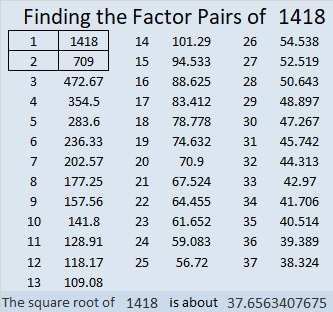# 1418 Challenge Puzzle

The 19 clues in this Find the Factors Challenge Puzzle are enough to find its unique solution. Can you find it?Print the puzzles or type the solution in this excel file: 10 Factors 1410-1418

Now I’ll write a few facts about the puzzle number, 1418:

• 1418 is a composite number.
• Prime factorization: 1418 = 2 × 709
• 1418 has no exponents greater than 1 in its prime factorization, so √1418 cannot be simplified.
• The exponents in the prime factorization are 1, and 1. Adding one to each exponent and multiplying we get (1 + 1)(1 + 1) = 2 × 2 = 4. Therefore 1418 has exactly 4 factors.
• The factors of 1418 are outlined with their factor pair partners in the graphic below.1418 is the sum of two squares:
37² + 7² = 1418

518-1320-1418 calculated from 2(37)(7), 37² – 7², 37² + 7².
It is also 2 times (259-660-709)

This site uses Akismet to reduce spam. Learn how your comment data is processed.